# 11. Safety evaluation for trains exposed to strong winds

• RTRI clarified the characteristics for aerodynamic forcesm strong winds and vehicle dynamics of vehicles subject to cross winds , and established a technique for quantitative evaluation of safety.
• These results can be utilised in hardware and/or software countermeasures for strong winds.

## [Dynamic characteristics of vehicles]

Research after the Amarube accident of 1986 demonstrated that the aerodynamic forces acting on vehicles depend on vehicle shape, the configuration of railway structures such as bridges and embankments, and the angle between the running vehicle and the wind (i.e. wind direction).

RTRI examined factors relating to these aerodynamic variables and vehicle dynamics, and proposed a detailed equation to calculate the critical wind velocity for overturning. This equation gives a limit wind velocity based on the static balance of forces acting on vehicles in the same way as the conventional Kunieda formula, but differs from the latter in its use of aerodynamic forces and center of gravity displacement (Table 1).

In addition, a simulation program was developed to extending the detailed calculation equation to dynamic analysis was prepared, and its adequacy was confirmed by a verification test using actual vehicles (Fig. 1). The results of the equation and dynamic analysis were also compared and studied with a view to their application for actual cases. In this way, a technique was proposed to enable the critical wind velocity for overturning to be evaluated by adding the effect of a vehicle’s dynamic characteristics to the detailed calculation equation.

## [Characteristics of aerodynamic forces acting on vehicles]

RTRI carried out wind tunnel tests on various vehicles and railway structures to evaluate the aerodynamic forces acting on vehicles (side force, lift, rolling moment). Table 2 shows one example, and the aerodynamic force coefficient can be estimated by selecting similar vehicle configurations or railway structures from the table to correspond to the shapes of the actual objects.

Each estimated aerodynamic coefficient can be used to calculate the critical wind velocity for overturning.

•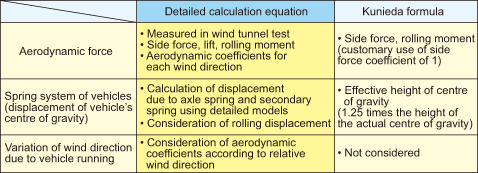Table 1 Main differences between the detailed calculation equation and the Kunieda formula
•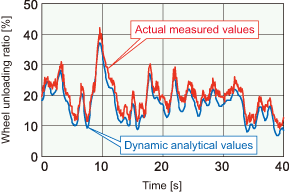Fig. 1 Verification by dynamic load testing of lateral forces
•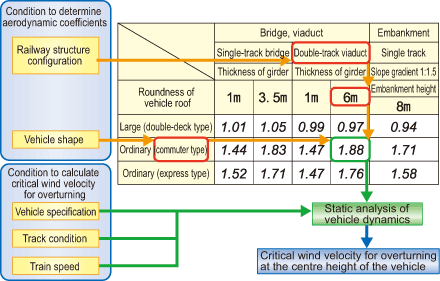Table 2 Example of aerodynamic coefficients

## [Strong wind characteristics]

The RTRI calculated the relationship between wind velocity measured using an anemometer near railway structures such as bridges/embankments and wind velocity at the evaluated position of critical wind velocity for overturning determined using the detailed calculation equation (wind velocity ratio of maximum instantaneous wind velocity) based on the results of wind tunnel testing and on-site observation (Fig. 2). This wind velocity ratio can be used to estimate the wind velocity at the evaluated position of critical wind velocity for overturning.

The frequency of rapid increases in wind velocity over a short time was also isolated as a statistical characteristic value from wind observation data for various regions. This value can be used to quantitatively evaluate the probability that the wind velocity acting on a train running in a regulated section will exceed a certain level.

## [Safety evaluation]

The RTRI established a technique to analyse tolerance in strong winds depending on differences in the specifications of vehicles, tracks, etc. based on a knowledge of the characteristics of dynamics, aerodynamics and response of vehicles to strong winds. The technique can be used to indicate the probability that a train running in a regulated section will encounter a wind velocity that exceeds the critical speed for overturning (which occurs due to rapid increases in wind velocity). This probability is proportional to the frequency of trains operating over a section, and is calculated based on the probability of strong winds along the railway line, the regulated wind velocity, the passage time in the regulated section, and the wait-and-see time until the resumption of operation.

This index allows the effects of strong winds on vehicle running to be quantitatively evaluated. By way of example, it makes it possible to investigate whether or not safety will be negatively influenced if the wind velocity level that triggers a notification to slow down is lowered when inhibited wind velocity is alleviated. At the same time, the effects of countermeasures, such as slowing down and windbreak fences can be determined to establish a safety level equivalent to that of advantageous specifications even if the specifications of vehicles and tracks are disadvantageous in relation to the critical wind velocity for overturning (Fig. 3).

•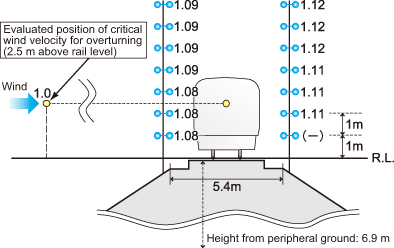Fig. 2 Example of the ratio of maximum instantaneous wind velocity at the position of an anemometer when the wind velocity at the evaluated position of critical wind velocity for overturning is set to 1.0 (Embankment case: The wind direction is 90° against the track.)
•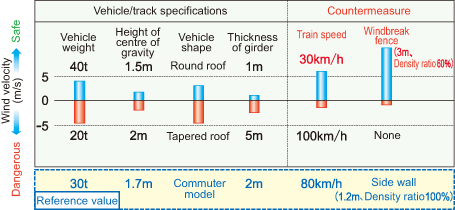Fig. 3 An example of evaluating the influence of vehicle/track specifications and countermeasures against the critical wind velocity for overturning (The level of influence is shown by the extent of the increase or decrease in the critical wind velocity for overturning.)